#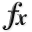N-D Test Functions Z¶

class go_benchmark.Zacharov(dimensions=2)

Zacharov test objective function.

This class defines the Zacharov global optimization problem. This is a multimodal minimization problem defined as follows: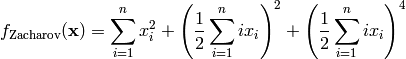Here,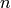represents the number of dimensions andfor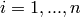.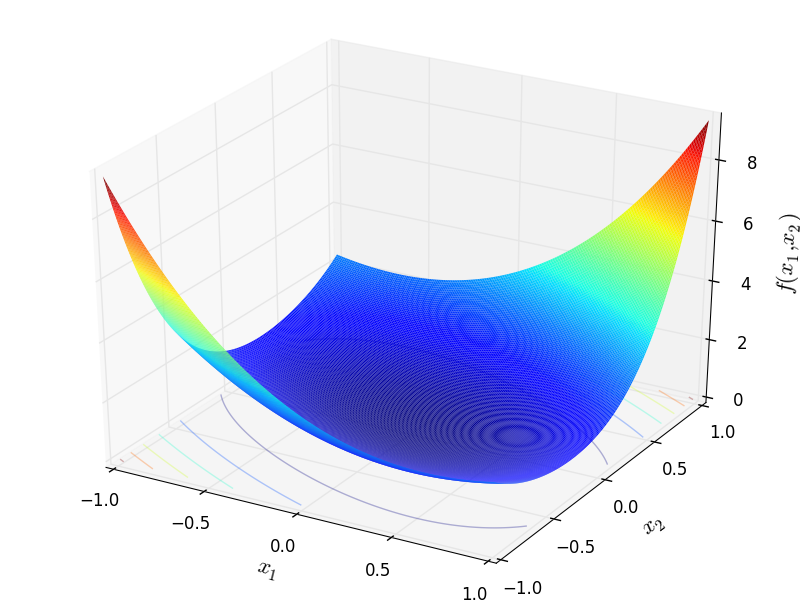Two-dimensional Zacharov function

Global optimum: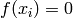for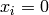forclass go_benchmark.ZeroSum(dimensions=2)

ZeroSum test objective function.

This class defines the ZeroSum global optimization problem. This is a multimodal minimization problem defined as follows: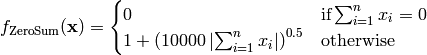Here,represents the number of dimensions andfor.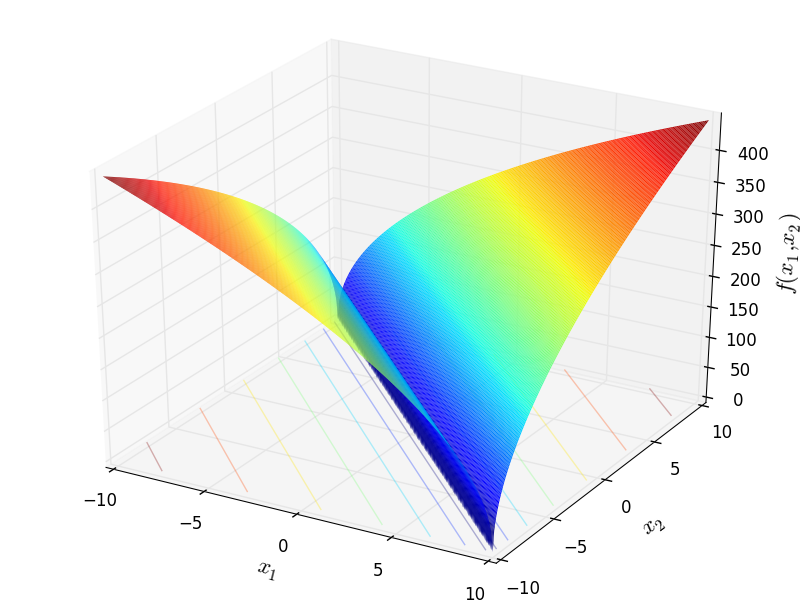Two-dimensional ZeroSum function

Global optimum:where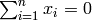class go_benchmark.Zettl(dimensions=2)

Zettl test objective function.

This class defines the Zettl global optimization problem. This is a multimodal minimization problem defined as follows:Here,represents the number of dimensions and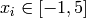for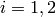.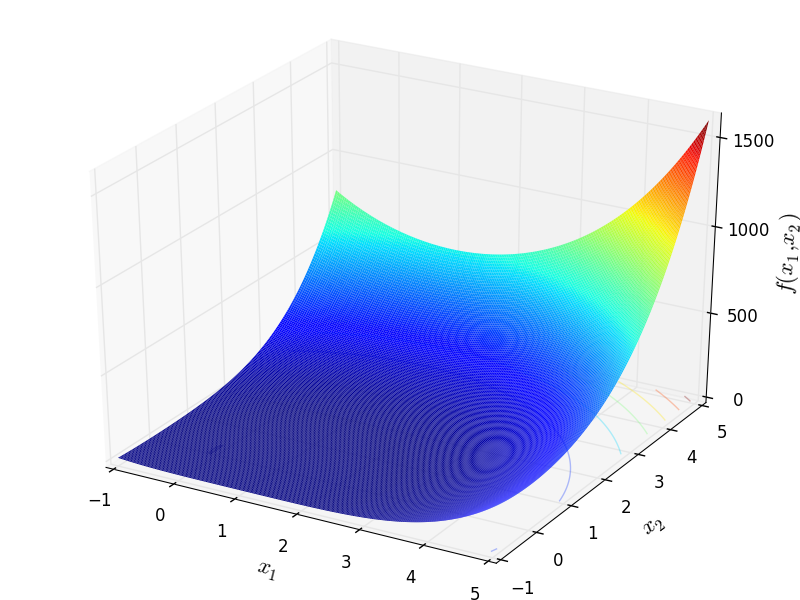Two-dimensional Zettl function

Global optimum:for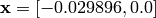class go_benchmark.Zimmerman(dimensions=2)

Zimmerman test objective function.

This class defines the Zimmerman global optimization problem. This is a multimodal minimization problem defined as follows: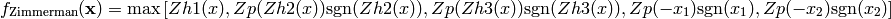Where, in this exercise: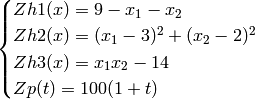Where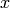is a vector andis a scalar.

Here,represents the number of dimensions and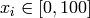for.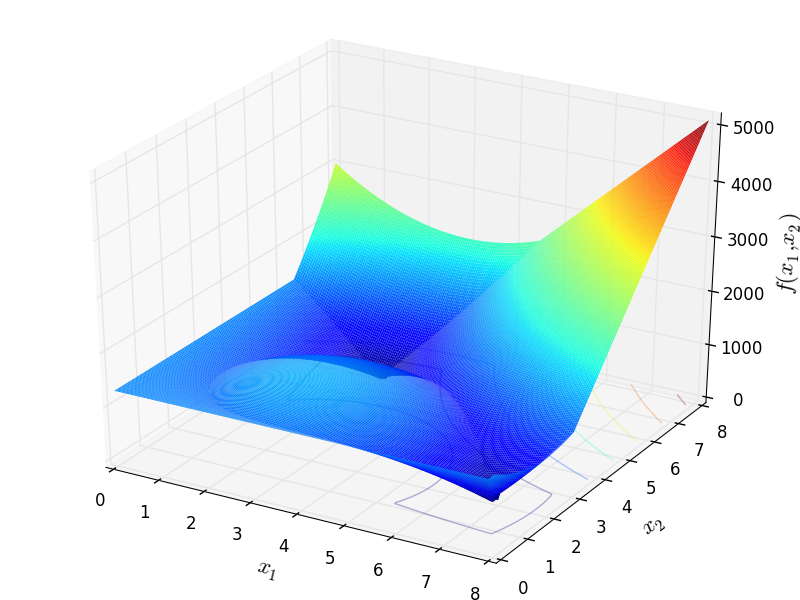Two-dimensional Zimmerman function

Global optimum:for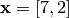class go_benchmark.Zirilli(dimensions=2)

Zettl test objective function.

This class defines the Zirilli global optimization problem. This is a unimodal minimization problem defined as follows: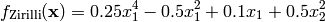Here,represents the number of dimensions andfor.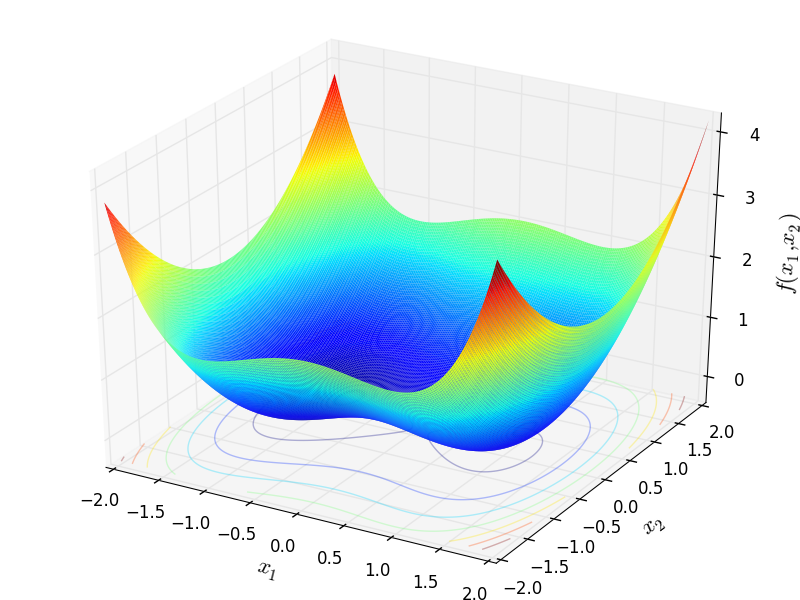Two-dimensional Zirilli function

Global optimum: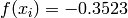for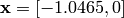#### Previous topic

N-D Test Functions Y

#### Next topic

1-D Test Functions